﻿ Application of Power Method and Dominant Eigenvector/Eigenvalue Concept for Approximate Eigenspace Solutions to Mechanical Engineering Algebraic SystemsPublications are Open
Access in this journal
Article Versions
Export Article
• Normal Style
• MLA Style
• APA Style
• Chicago Style
Research Article
Open Access Peer-reviewed

### Application of Power Method and Dominant Eigenvector/Eigenvalue Concept for Approximate Eigenspace Solutions to Mechanical Engineering Algebraic Systems

Michael J. Panza
American Journal of Mechanical Engineering. 2018, 6(3), 98-113. DOI: 10.12691/ajme-6-3-3
Received September 11, 2018; Revised October 14, 2018; Accepted November 14, 2018

### Abstract

This paper shows how the concept of dominant eigenvector/eigenvalue and the power method can be used for understanding the solution to practical problems in a broad class of mechanical engineering systems described by linear algebraic equations. An analytical mathematical procedure is developed to obtain a reasonably accurate approximate eigenspace solution to the system Ax=b by transforming the non-symmetric system matrix into a form where the power method is used several times to compute the contribution of only one or two eigenvector/eigenvalue pairs that dominate the solution. The complete set of eigenvalues and eigenvectors is not required. The intent is to provide a novel application of and show the importance of the significance of dominant eigenvector/eigenvalue pairs and to use the power method in the analysis and understanding of mechanical engineering systems for both education and practice. Typically, the concepts of both eigenvector expansion and dominant contribution are not included in mechanical engineering education. The scope of application is a broad area of six practical mechanical engineering problems including translational and rotational dynamics, statics of structures, thermal energy balance, fluid continuity, and feedback control. These general mechanical engineering systems naturally contain numerical A and b matrices that fit the scope suitable for providing feasible accuracy. The systems range from fourth order to two hundredth order. Results indicate that accuracy greatly improves as the A matrix contains elements of the same order of numerical magnitude, either naturally from the physics of the problem, or through a transformation to dimensionless parameters. Motivation for using the power method for dominant eigenvector/eigenvalue calculation comes from methods used in web page rank algorithms and a desire to expand mechanical engineering students’ education in understanding the role of the eigenstate expansion method for the solution of algebraic equations without computing all of its eigenvalues and eigenvectors. An in depth quantitative assessment of the approximate dominant eigenspace method accuracy is obtained by testing the mechanical engineering examples against a near exact solution via a software solver. This error assessment is based on parameters useful in the design and understanding of mechanical systems. The accuracy achieved is feasible for education and for other uses of the method by practicing mechanical engineers such as when a simple analytical based approximate model may be more suited for inclusion into larger system based models.

### 1. Introduction

For mechanical engineering professionals and students, there are several excellent numerical methods for solving a system of linear algebraic equations including based on Gaussian elimination. These are thoroughly discussed in books on matrix methods for engineers 1, 2, 3, 4 and advanced engineering mathematics 5, 6, 7. Also in these books are methods for finding all of the eigenvalues and eigenvectors of a matrix. However for systems of linear algebraic equations in mechanical engineering that are numerous in static structures, dynamic machines, steady state thermal/fluid systems, and biomechanics, very little interest or work has been seen in these books or in the literature about the use of eigenspace expansion representation for the solution to Ax=b. For engineering design and analysis, the role of eigenvalues/eigenvectors of system matrix A and the solution x can be very useful for student learning of the underlining engineering concepts or an engineer’s understanding of system behavior. Showing the importance of the role of eigenvalue/eigenvector pairs is a motive for the work presented here. The motive is also to show that the lower frequencies of eigenvalue/eigenvector pairs dominant the solution of mechanical engineering systems and how the power method can be applied to determine the effect of these lowest two eigenstates to form an accurate approximate solution for Ax=b without calculating the complete eigenstate of A. The intent of the proposed dominant eigenvalue, eigenvector method is not to obtain the near exact solution of Gaussian based numerical solvers but to obtain an accuracy that may be considered suitable so that an approximate method may be useful for understanding the role of the lower eigenstates and for use in larger analysis models like engineering design and optimization of system parameters where a compact analytically based simpler method is desirable for overall computational efficiency.

The use of eigenvector expansion as a way of representing the solution of systems has received a great deal of attention for systems of second order ordinary differential equations in the areas of mechanical and structural vibrations. Two notable texts 8, 9 show when the system matrix is symmetric or can be made so via a simple transformation, an orthogonal set of eigenvectors can be used to represent the vibration response. The orthonormal eigenspace leads directly to determining the contribution of each eigenvector independent of the others. This is explained in the mathematical model. The eigenspace representation for the solution to the systems of ordinary differential equations provides insight with respect to how various system modes of vibration affect the solution and allow its control. Recent applications to several current practical mechanical engineering vibration problems include predicting the human induced vibration of foot bridges for crowd–structure interactions 10, the vibration of armature-receiver in hearing aids using methods of mode decomposition, truncation, and selection 11, and the problem of finding the optimal stiffness and damping coefficients of a two degree of freedom system acting as a dynamic vibration absorber 12. All of the systems in [8-12] 8 are based on second order differential equations.

Very little interest or work has been seen in the literature or books about the use of eigenspace expansion representation for the solution to Ax=b. Buckling of a beam was analyzed with eigenvector expansion 13 but little other work has been published. A notable exception is a set of supplemental notes used in an undergraduate math class 14 where the eigenvector expansion technique for linear algebraic systems is clearly presented.

The power method for determining the dominant eigenvector and eigenvalue of a matrix is clearly presented in 15. In 16 a ranking methodology which may be of interest in some sports results analyses is introduced and investigated by applying a dominant eigenvector with power method concept to a matrix model. Arguably one of the most noteworthy applications of the dominant eigenvector concept to matrix analysis is the Google page rank algorithm given in 17. The work in 18 provides additional clarity on the use of elements of the dominant eigenvector of a matrix to represent the rank of web pages. Also, 15 gives an excellent description of the Google page rank algorithm. The web page ranking method in 15, 17, 18 is based on setting up a square stochastic matrix with maximum eigenvalue equal to one from a large labyrinth of possible web pages related to a particular search statement and the connection of associated web pages. The dominant eigenvector associated with it is computed with a power method based procedure and its elements correspond to ranking values of the webpages pages used to develop the matrix.

This work presented in this paper is motivated by the above focusing on the use of the dominant eigenvector concept to investigate the behavior of and obtain meaningful results for a system modeled with a square matrix without having to compute any other eigenvectors or eigenvalues of the system matrix. Motivation also comes from a desire to provide a good example of eigenvector/eigenvalue application to mechanical engineering students by including dominant eigenvector/eigenvalue calculation via the power method. Such an application can be expected to broaden student’s appreciation of eigenvector/eigenvalue analysis and the role dominant eigenvectors play in understanding the behavior of small to large algebraic systems so common in mechanical engineering. The concept of dominant eigenvector/eigenvalue and the power method are combined with the technique of eigenvector expansion for solving practical problems in mechanical engineering described by systems of linear algebraic equations. This represents an extension of the web page ranking concept developed for a special type of matrix to more general non-symmetric matrices that typically result from the application of physical principles used in the modeling, analysis, and design of mechanical engineering systems. Also, an extension is made from a dominant eigenvector determination of one matrix to the solution of a complete set of algebraic equations. An analytical mathematical procedure is developed to obtain an accurate approximate eigenspace solution to the system Ax=b by transforming the system into a form where the power method can be used to accurately compute the contribution of the eigenvector/eigenvalue pairs of the smallest two eigenvalues. Motivation for calculating only the eigenvector/eigenvector pair for the lowest two eigenvalues requires an A matrix numerical element check and possible transformation into dimensionless form so that the elements of the A matrix for which the method is applied has element values on the same order of magnitude.

The goals and scope of the work is given as follows:

• Develop an analytical method based on several applications of the power method for determining the eigenvector/eigenvalue pairs of the lowest two eigenvalues of a matrix associated with a general non-symmetric system matrix that generally occurs in mechanical engineering linear algebraic systems. The method does not require determination of any eigenvector/eigenvalue pair for eigenvalues greater than the lowest two.

• Provide a novel application of and show the importance of the dominance of the lowest two eigenvalue eigenvector/eigenvalue pairs in an eigenspace expansion representation and use of the power method in the analysis and understanding of mechanical engineering systems for both education and practice. Typically, the concepts of both eigenvector expansion and dominant contribution for linear algebraic systems are not directly included in mechanical engineering education and analysis.

• Develop an approximate method that transforms the general non-symmetric system matrix A in mechanical systems to a suitable symmetric, well-conditioned form for several applications of the power method for determining the eigenvevtor/eigenvalue pairs of the smallest two eigenvalues.

• Study the accuracy of the approximate method for a broad area of six mechanical engineering problems ranging from fourth order to two hundredth order including translational and rotational dynamics, statics of structures, thermal energy balance, fluid continuity, and feedback control. These mechanical systems encompasse practically all mechanical engineering principles and always have an input vector b that contains at least 50% zero elements.

• Show the pros and cons of the approximate method by comparing its results for using the lowest or the lowest two dominant eigenvector/eigenvalue pairs to an exact solution commonly obtained with a numerical solver.

• Provide an extensive quantitative assessment of error between approximate method and exact solution by testing it against a software solution including the significantly small effect of eigenvector/eigenvalue pairs above the two smallest eigenvalues to demonstrate a feasibility level and confidence for using the approximate closed form method. The goal is to provide a high degree of confidence that for typical mechanical engineering systems, eigenvector/eigenvalue pairs above the lowest two eigenvalues are insignificant relative to the intended use of the approximate model.

• Outline an approximate method that can stimulate and encourage both mechanical engineering students and practicing mechanical engineers to understand the significance of the dominant eigenvector/eigenvalue pairs and have confidence for use in larger analysis models like engineering simulation, design and optimization of system parameters where a compact analytically based simpler method is desirable for overall computational efficiency. For example, a complete system model of the complex system of diesel engine cooling such as in a locomotive or other transportation vehicle is composed of a complex mixed discipline system of the principles of thermodynamics, heat and mass transfer, fluid mechanics, valve mechanical mechanisms, and control schemes. In the steady state, the A matrices for the sub-systems are coupled together and each can contain orders in the single digits to the hundreds. The overall system model can be enormous and be computationally inefficient for just one simulation let alone when used in an optimization model. Simpler dominant eigenspace models for the sub-systems may provide both improved computational efficiency and allow one to more clearly identify problem areas and design changes that can be made and control schemes that can be designed to meet cooling requirements. Other examples of large systems may come from areas such as biomechanics and robotics where mixed principle systems are important and simpler subsystem models are required to design control schemes.

• The intent of the approximate method is not to provide an alternate solution to Ax=b for any order and any type of matrices A and b that one may be using. The only application investigated here is for a broad class of actual mechanical systems of order four to two hundred derived from mechanical engineering principles which mostly always meet or can be transformed to meet the requirements of a system A matrix with elements of the same order of magnitude and an input B vector with over fifty percent zero elements. It is these type of systems that best fit the model where the eigenvector for the lowest and/or the second lowest eigenvalue can be expected to provide an accurate and feasible solution to show the use of the eigenspace expansion to engineering students and for potential use in the analysis complex mechanical engineering system models. This may also encourage one to apply the approximate method to systems form other disciplines that meet these requirements.

### 2. Mathematical Procedure

An approximate solution for the vector x in the nth order linear algebraic system Ax=b to an input vector b is developed by using an eigenspace expansion approach and applying the power method to determine the dominant eigenvector and dominant eigenvalue of matrices associated with the system matrix A. The direct calculation approach using Gaussian elimination is a very accurate solution but may not provide enough information and insight into system behavior as a method based on eigenvectors and eigenvalues may provide.

The dominant eigenvector of a matrix is the eigenvector typically associated with largest eigenvalue (in the absolute value sense) of the matrix. The power method provides the dominant eigenvector and then the Rayleigh quotient may be used to find dominant eigenvalue. For the problem of finding the input b required to provide a particular desired output x, direct application of the power method to matrix A can be used to develop a reasonable approximate solution due to dominance in b generally coming from the largest eigenvalue of A. For the problem proposed above for finding output x for a specified input b, it generally is the smallest eigenvalue of A that dominates in x.

Given a general Nth order non-symmetrical square matrix A that is commonplace in mechanical engineering systems, the approach is to transform Ax=b into a system where the application of the power method to a new transformed matrix will lead directly to an approximate solution related to the smallest eigenvector/eigenvalue of A. This approach requires developing a positive definite symmetrical matrix E from matrix A.

To obtain a system with positive definite matrix E that can be used to provide an approximate solution to the system Ax=b, where A is invertible, first make the transformation x=ATz giving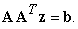(1)

Defining the resulting symmetrical positive definite matrix E=AAT and using a special procedure for calculating the smallest eigenvector/eigenvalue of E by applying the power method and Rayleigh quotient a couple of times, is shown that an approximate solution for z leads to an approximate solution for xa given by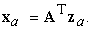(2)

If the system matrix A is a symmetric positive definite matrix, then E=A is used for the analysis.

Consider the system(3)

where E is a positive definite symmetric regular matrix which will have real positive eigenvalues λEn and orthonormal eigenvectors vEn Expanding z in the eigenspace of E with the real scalar set of coefficients cn gives(4)

Substituting into eqn. (3) gives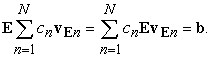(5)

Substituting the eigenvector form EvEn= λEnvEn in eqn. (5) gives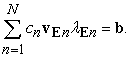(6)

Multiplying both sides of Eqn. (6) by the transpose of a single eigenvector (vE j)T gives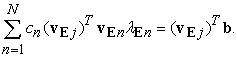(7)

Orthonormal eigenvectors give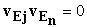for j ≠ n and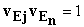for j = n and thus all terms in the sum are zero except for j=n. Thus each cn can be determined independently as(8)

In this paper, the approximate model is considered for the smallest eigenvalue of E or for the combination of the two smallest eigenvalues of E. As equation (8) shows that cn is inversely proportional to eigenvalue, significant contributions may come from the smaller eigenvalues and dominate the solution as long as the inner product (vEn)Tb of the numerator is not very large for a higher eigenvalue. The numerator indicates that the closer the match of direction for (vEn) and b, the larger the value. The approximate method of one or two dominant eigenvalue/eigenvector pairs counts on a low probability of a good match. The nature of the mechanical engineering problems examined is that there are generally over fifty percent zero elements in b. In fact, most mechanical systems have only a few inputs. (the examples used here cover a broad range of mechanical engineering systems having 50% to 99% zeros in b). Thus due to this mismatch and the reciprocal eigenvalue contribution, it is very unlikely that eigenvalue/eigenvector pairs higher than two would have a c value large enough to significantly affect the intended accuracy of the approximate model. Additionally, transforming the A matrix obtained from physical principles into one where the numerical of ratio of the largest to the smallest element is small, say on the order of ten or less is an important part of the procedure to provide good accuracy. This defines the scope of the problems that the approximate model developed in this paper is useful for. It will be shown that over the wide range of examples presented, fair accuracy from 5 to 39% is obtained with the smallest eigenvalue and improved to 1 to 13% by adding the second eigenvalue. This is feasible for using the model as an approximate analysis tool, as a means of understanding system behavior relative to design, and for confident use in a larger mathematical model for situations such as optimization. The goal of the model is to calculate the lowest and second lowest eigenvalue/eigenvector pair by several applications of the power method without computing all of the eigenvalue/eigenvector pairs. This can serve as a useful addition of eigenvalue/eigenvector application in engineering analysis education and for practicing engineers.

For the symmetric positive definite matrix E, all of the eigenvalues are positive. To determine an approximate solution to eqn. (1) from the smallest eigenvalue of E, we start with applying the power method to E to determine its dominant eigenvector vEd and its norm vEdn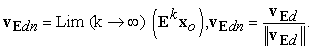(9)

The Lim (k→∞) in eqn. (9) is defined as the iteration of k where convergence to three decimal places is reached.

Applying Rayleigh’s quotient to determine the largest eigenvalue λE max gives(10)

To determine the smallest eigenvalue of E and the eigenvector associated with it, set up a matrix(11)

where I is a N by N identity matrix.

Applying the power method toto determine its dominant eigenvector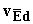and its norm(12)

Applying Rayleigh’s quotient to determine the largest eigenvalue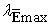gives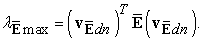(13)

Since E is positive definite, λE max is positive and with the definition of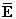in eqn. (11),will be negative and then λE min is given by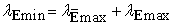(14)

λE min andare expected to provide the primary contribution of the solution to Ez=b.

From eqns. (2) and (8), the n=1 term is the exact contribution from the smallest eigenvalue of E and is defined as the approximate solution to Ez=b due to λE min and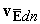. It is given by(15)

To obtain an approximation for second smallest eigenvalue of E,is deflated withand.(16)

Applying the power method to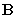to determine its dominant eigenvector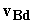and its norm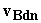gives(17)

Applying Rayleigh’s quotient to determine the largest eigenvaluegives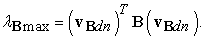(18)

The second smallest eigenvalue of matrix E is given by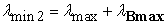(19)

whereis the largest eigenvalue of E andis the eigenvector for the second smallest eigenvalue of E. As in equation (15), the exact contribution from the n=2 term is given by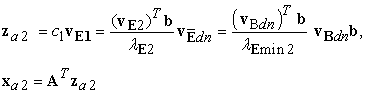(20)

The numerical examples will compare xa=xa1 from equation (15) and xa=xa1+ xa2 from equations (15) and (20) to the exact direct solution of Ax=b obtained via Gaussian elimination.

### 3. Applications

Several applications in the general field of mechanical engineering are examined. The purpose is to explore the following aspects related to the breadth and accuracy of the approximate method developed in section two. These aspects define the scope of applicability for the approximate method.

a. Examples are for a wide range of steady state models algebraic of systems described by Ax=b in the following areas of mechanical engineering obtained from physical principles including static equilibrium, Newton’s laws of motion, Euler’s equations of rotation, first law of thermodynamics, Fourier’s law of heat conduction, and conservation of mass. The order of the A matrix for the examples range from four to four hundred. All of the examples have 50% or more zero elements in the input vector b as is typically the case for mechanical engineering system of order four or more. The particular percentage of zeros in b are given in parentheses.

1. 4th order classical dynamics of blocks on an inclined plane (50%)

2. 7th order mechanism with translational and rotational inertial elements. (57%)

3. 12th order statics of a structural frame. (67%)

4. 5th order closed loop control of a system with the interaction of thermodynamics, heat transfer, and fluid mechanics. (60%)

5. 6th order biomechanics of human arm motion. (67%)

6. 6th to 400th order chain of N mass and dissipated elements (83% to 99%)

b. Systems of various orders from four to a four hundred.

c. Various parameter values for a particular system.

d. Transformation to dimensionless parameters to provide an A matrix with a reasonably small numerical ratio of its largest to smallest element to improve approximation accuracy.

3.1. Example 1: Classical Dynamics of Blocks on an Inclined Plane

Figure 1 A is a schematic of blocks on an inclined plane connected by a pulley system. The dynamic problem is to determine block acceleration aB, and cable tensions TA, TB, and TC just after the system is released from rest. Coefficient of dynamic friction The fourth order matrix, vector dynamic equations from Newton’s laws are given by(21)

The following numerical example is used: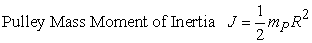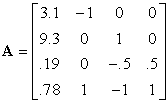(22)
• Figure 1A. Schematic of Inclined Plane Dynamic System

Due to the combination of acceleration and force coefficients, there is a wide range for elements of the A matrix. The ratio of the largest element to smallest element of A is 49. To improve the scaling to get elements that are of similar order of magnitude and improve the approximation, consider a transformation from the physical states to dimensionless stares and parameters. The application of scaling and dimensionless representations was explored in 19, 20, 24 for a few specific algebraic and differential equations occurring in simple fluid systems. Detail investigations of how transformation to dimensionless variables and parameters lead to system matrices containing far fewer dimensionless parameters than the number of real parameters in the original matrix developed from applying physical principles are given in 21, 22, 23. These three papers are concerned with complex systems that include the interaction of electrical and hydraulic actuators with the dynamics of mechanisms and structures. The cited references 19, 20, 21, 22, 23, 24 provide details on how the dimensionless systems used here are derived. Basically, the approach is to first divide all of the force, moment equations obtained from physical principles by an important physical parameter in the problem, such as the weight of a mass. This brings in the acceleration due to gravity g into the equations. Then the dimensionless forces and accelerations are defined relative to this one mass and g. The coefficients of the equations then become dimensionless parameters that are defined as dimensionless mass ratios or rearranged as a combination of a couple of other real parameters to produce dimensionless coefficients

For the inclined plane example, the A matrix is transformed by using the following dimensionless description(23)

With KB=0.67, KP=0.25, the numerical dimensionless A matrix is given as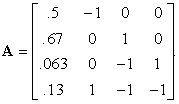(24)

Now the coefficients are of the same order of magnitude with the ratio of largest to smallest element being 10. For Figures 1B through 6B, the horizontal axis is the state number, which is the element number of the exact and the approximate vectors. The vertical axis is the value of that element in the exact vector x (Gaussian software solution to Ax=b) and approximation xa (approximate solution using the one or two dominant eigenvector model).

Figure 1 B compares the approximate solution from equations (15) and (20) based on the one and two dominant eigenvector/eigenvalue approximate model to the exact solution obtained from a software solver. It clearly shows that dimensionless system model leads to improved accuracy. The primary part comes from the lowest frequency response where the approximation has a good trend match with the exact solution indicating dominance of the lowest frequency eigenvector/eigenvalue pair. Including the second lowest frequency approximation provides additional accuracy. This example is used as a guide for when to apply dimensionless analysis to matrix A with real dimension elements to provide a dimensionless A with dimensionless elements. A general rule of thumb is to scale A such that an element ratio of less than about 10 is obtained.

To further demonstrate how the eigenvector/eigenvalue pair for the lowest eigenvalue of E provides the primary part of the solution for this mechanical system relative to an additional accuracy correction from the second lowest pair and the potential very small amoutst from higher frequencies, and to justify the discussion after equation (8) asserting that due to the fact that since the input vector b contains fifty or more percent zero elements making it unlikely that higher frequencies will have a good enough match of eigenvector to input vector to overcome the reciprocal frequency effect to cause dominance other than the two lowest, the inner product and coefficient of all eigenvector/eigenvalue pairs are calculated with a software solver and compared in Table 1. Note that the inner products are of the same order of magnitude, primarily due to the greater than fifty percent zeros in b, allowing the reciprocal eigenvalue to control cn.

3.2. Example 2: Mechanism with Translational and Rotational Inertial Elements
• Figure 2A. Schematic of Dynamic Mechanism

Figure 2 A is a schematic of a mechanism composed of a block and disc connected by a pulley system. The dynamic problem is to determine block acceleration aB and disc acceleration aA disc friction force FA, and cable tensions TA, TB, TC, TD just after the system is released from rest. The seventh order matrix, vector dynamic equations from Newton’s laws are given by(25)

The following numerical example is used:(26)

As for the inclined plane example 1, due to the combination of acceleration and force coefficients and the combined application of Newton’s and Euler’s laws, there is a wide range for elements of the A matrix. The ratio of largest element to smallest element of A is 200. To improve the scaling and thus the approximation, consider a transformation from the physical states to dimensionless stares and parameters given by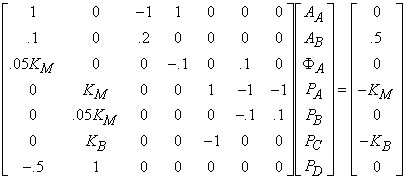(27)

With KM=0.75, KB=0.5, the numerical dimensionless A matrix is given as(28)

Now the coefficients are of the same order of magnitude with a max to min element ratio of 8.

Due to the inclined plane example 1 being much more accurate with the dimensionless A, example 2 is only presented as a dimensionless model. Figure 2 B compares the approximate solution based on the one and two dominant eigenvector/eigenvalue pair to the exact solution obtained from a software solver. As is the case for the inclined plane example 1, it clearly shows that the dimensionless system has good accuracy.

For example 2, the second lowest eigenvalue is an integral part of the approximate model.

For examples one and two, the dimensionless models are more accurate in that the numerical dimensionless values of inputs, outputs, and parameters are typically in a somewhat narrow range of values. The values for inputs, outputs, and parameters in the physical models are typically in a wide range due to the different physical units that result from the combined application of Newton’s second law of motion for translation and Euler’s law of rotation. This apparently provides some advantage for using dimensionless models to have a suitable A matrix for this approximation procedure.

Table 2 further demonstrates how the lowest two eigenvector/eigenvalue pairs of E provide the primary part of the solution for this mechanical system.

For this mechanism example, the third lowest frequency’s large c3 value is an oddity and the inclusion of the third frequency component does not provide any significant improvement for the states 5, 6, and 7 which have the highest errors for the two frequency approximate model, which results in s reasonably low average error across states as will be shown in an error analysis after presentation of all six examples.

3.3. Example 3: Statics of a structural frame

Figure 3 A is a schematic of a structural frame. The static equilibrium problem is to simultaneously determine the four external support reactions R1, N1, R2, N2 and the eight frame internal forces A through H when subjected to external forces F2=2 at joint 2 and F4=1.4 at joint 4. The twelfth order matrix, vector equations from static equilibrium are given by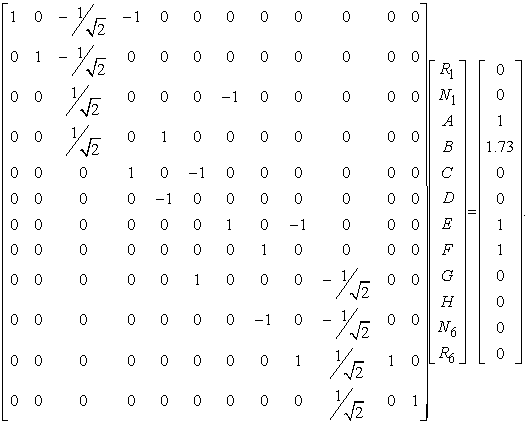(29)
• Figure 3A. Schematic of a structural frame in equilibrium

Figure 3 B compares the approximate solution from equations (15) and (20) based on the one and two dominant eigenvector/eigenvalue approximate model to the exact solution obtained from a software solver. The approximate solution follows a very similar shape as the exact solution. This close shape trend indicates that the dominant eigenvector is a key factor in the solution. One reason for this is that one physical principal provides all of the equations and also for this case, the elements of A are from trigonometry which are dimensionless and thus coefficients tend to be naturally of the same order of magnitude. The ratio of max to min element value in A is 1.5 indicating that elements are of the same order of magnitude. Accuracy across the twelve states is reasonable.

Table 3 further demonstrates how the lowest eigenvector/eigenvalue pair of E provides the primary part of the solution for this mechanical system with the second pair adding only a small variance.

3.4. Example 4: Closed Loop Control of a System with the Interaction of Thermodynamics and Fluid Mechanics
• Figure 4A. Schematic of a Thermal-Fluid System with Feedback Control

Figure 4 A is a schematic of a thermal fluid control system for regulating the temperature Ts of an air space. Variable designations are T’s (temperature), p’s (fluid pressure), and Q’s (fluid volume flow). Physical parameters are R’s (thermal and fluid resistance), KT,p (feedback control gains), and fluid/thermal heat conversion Kh. Application of thermodynamic equilibrium for the air space and container walls give two equations. Application of fluid statics for the two flow lines gives the next two equations. Application of fluid continuity and fluid to thermal transfer for the heater gives a fifth equation. Feedback control is included in the five equations as required. Thus the five equations contain coefficients from several physical principles. Following the results from examples one and two where the A matrix contains elements resulting from several physical phenomena, the following dimensionless states xi and parameters Ki are defined to provide an A matrix with elements being of the same order of magnitude.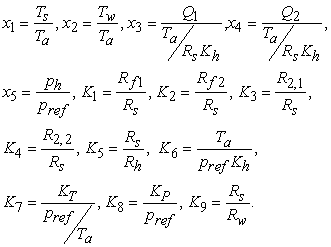(30)

The dimensionless inputs are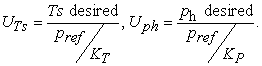The thermal fluid problem is to determine the steady state temperature, flow, and pressure, The fifth order matrix, vector dimensionless equations are given by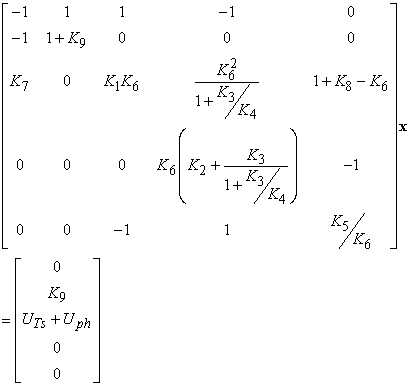(31)

Figure 4 B compares the approximate solution based on dominant eigenvector, eigenvalue to the exact solution obtained from a software solver. The values UTS=1 and Uph=0.5 are used. The approximate solution for both the K value sets of all Ki=1 and for K randomly selected from a uniform distribution from K=.25 to K=4 very accurately represent the exact solution for all five states. The elements of the A matrix are of the same order of magnitude for this practical range of K values, e.g. for all K’s=1, the ratio of max to min element of A is 4. The approximate lowest frequency solution follows a very similar shape as the exact solution. This close shape trend indicates that the dominant eigenvector is a key factor in the solution. For this example, the contribution of the second smallest eigenvalue is minimal.

Table 4 (for all K’s=1) further demonstrates how the lowest eigenvector/eigenvalue pair of E provides the primary part of the solution for this mechanical system with the second pair adding only a small variance.

3.5. Example 5: Biomechanics of Human Arm Motion

Figure 5 A is a schematic of a two link model of human arm biomechanics with A being the shoulder joint and B the elbow joint.. The dynamic problem is to determine the upper and forearm angular accelerations α1, α2, elbow joint forces Bx, By, and the accelerations acx, acy of the forearms center of gravity just after the system is released from rest. The sixth order matrix, vector dynamic equations from Newton’s and Euler’s laws and kinematic constraints are given by(32)
• Figure 5A. Schematic of Link Model for Human Arm

For a typical human arm at a location θ1=30 degrees, θ2=45 degrees,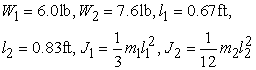(33)

For this example, even though the elements of the physical A matrix have different units, all non-zero elements other than two of them are between .24 and 1 and have a maximum ratio of 4. The other two have about a factor of ten ratio and both come from inertia terms. This is considered sufficient to expect good results without a dimensionless transformation.

Figure 5 B compares the approximate solution based on dominant eigenvector, eigenvalue to the exact solution obtained from a software solver. The approximate values of the elbow joint forces and forearm accelerations are very close to exact values while the approximate, exact comparison for the two arm angular accelerations are different by factor of 1.5 to 2 in the sense of absolute values.

Table 5 further demonstrates how the lowest two eigenvector/eigenvalue pairs of E provide the primary part of the solution for this mechanical system.

3.6. Example 6: Chain of N mass and Dissipated Elements

Figure 6 A is a schematic of N block masses connected by N dissipated damper elements bi and subjected to a force F1 at one end. The dynamic problem is to determine velocity VN of the last block. The Nth order matrix, vector dynamic equations from Newton’s laws are given by(34)
• Figure 6A. Schematic of Chain Mass-Damper Elements

Figure 6 B compares the approximate solution based on dominant eigenvector, eigenvalue to the exact solution obtained from a software solver for a specific set of six b values in a range of 2 to 6.Since all elements of A have the same dimension, they are of the same order of magnitude with a ratio of 5. Accuracy is good for all six states especially state six at the end of the chain.

Table 6 further demonstrates how the lowest two eigenvector/eigenvalue pairs of E provide the primary part of the solution for this mechanical system.

To summarize the accuracy over the six examples, Figures 1B-6B give a comparison of all states with a short qualitative assessment after each one. Since the intent is of the method is to understand system behavior and accuracy for design, a special quantitative error analysis may be based on the important matters pertaining to this intent. For these mechanical engineering systems, it’s the magnitudes of the states that are most important in design and behavior considerations. The magnitude the of variables for examples 1, 2, 3, 5, 6 are accelerations, forces, and displacements which lead to internal stress in system members and inertial forces that may lead to system support vibration. For example 4, the magnitude of variables temperature, flow, and pressure lead to system performance. Additionally, it is usually the magnitude of the largest variable that sets a standard for meeting design requirements. To quantitatively show how good the one eigenvalue, eigenvector method may be in regard to this criteria, the following error based on the ratio of the magnitude difference between approximate and exact states to the magnitude of the largest exact state is used.(35)

Table 7 gives an assessment of the accuracy of each state and an average over states for each example using the error in eqn. (35). Also included in Table 1 is the magnitude of the ratio of the largest to smallest element in the A matrix for each example to show how the makeup of the A matrix as containing elements with real units or dimensionless parameters affects the accuracy.

The results show that for A matrix conditioned such that max to min element ratios from 1.5 to 10, the overall average error of the states is 1% to 13% for the two eigenvector approximation over the entire range of the six examples. This level of error may be considered feasible for the previously discussed goals of the method. The accuracy of the results for the broad range of mechanical engineering problems allows both students and practicing engineers to use the two vector approximate method with confidence for both understanding the eigenspace concept and using the model in more complex systems analyses. The best cases are the dimensionless thermal, fluid dimensionless system of example 4 and the mechanical chain of example 6. The thermal system is a combination of the physical principles of thermodynamics, incompressible and compressible fluid flow and equations of feedback control. The mechanical chain is a repetitive application of one physical principle. For these two cases, the one vector approximation is sufficient. Furthermore, Table 1B-Table 6B indicate that inclusion of eigenvalues larger than the second lowest should not be of any significance relative to the goals of the approximate model. As one becomes more familiar with the nature of the mechanical system relative to one of the types analyzed here, one may become more confident with a one vector approximation but he results of these examples indicate that any doubt can be significantly reduced or essentially eliminated by using a two vector approximation. Additional confidence follows the fact that the six examples used here include all of the major physical principles that mechanical engineers deal with.

### 4. Application to Higher Order Example 6 Chains

Figures 6 C through 6 H compare the approximate value of the Nth state based on dominant eigenvector, eigenvalue to the exact value obtained from the matrix inverse by computing the error between approximate and exact values for ten sets of randomly selected bi values between 2 and 6. The figures give the Nth state error for a system of order 6, 20, 50, 100, and 200 states. The last state which is farthest from the input force is typically the most important for these train type of systems.

For figures 6C through 6H, the horizontal axis is number of the random set number of the six bi values, where there is a total of ten sets used. The vertical axis is the value of the percent error for the last state of a total of N states between the exact and approximate vectors x (exact solution to Ax=b) and xa (approximate solution using the one eigenvector, eigenvalue method). This error is defined as(36)

Table 8 gives a summary of the previous figures (6C-6G) with the average values of the last state error across all of the 10 random sets of b values for each of the N=6, 20, 50 100, 200 order systems.

The results show that the average error across random trials is typically in the range of 1to 8 percent for the one eigenvector model and 0 to 5 percent for the two eigenvector model. For the intent previously discussed, for this example 6 class of high order chain systems in mechanical engineering, the level of error may be considered to be reasonably low for such an approxmation that requires a simple power method based direct calculation of only one or two eigenstates of the system matrix rather than a more complex calculation of the entire eigenspace.

### 5. Conclusion

An analytical method is developed based on several applications of the power method for determining the eigenvector/eigenvalue pairs of the lowest two eigenvalues of a matrix associated with a general non-symmetric system matrix that generally occurs in mechanical engineering linear algebraic systems. The method does not require determination of any eigenvector/eigenvalue pair for eigenvalues greater than the lowest two. The present paper suggests a new simple analytical method of obtaining an approximate solution for the solution to a broad class of systems of linear algebraic equations in most of physical areas of mechanical engineering based on the power method for finding the dominant eigenvector and eigenvalue of matrices. The method includes a view of the system matrix A and input vector b and a possible transformation to a symmetric system and/or a dimensionless form to provide a system that is well conditioned to the proposed method of eigenvector expansion for the lowest two eigenvalues. The power method is applied several times to a symmetric matrix obtained from the conditioned A matrix to determine the eigenvector/eigenvalue pair for the lowest two eigenvalues. For engineering design and analysis, this approximate solution can be very useful for student learning of the role of dominant eigenvector/eigenvalue in the solution of algebraic systems and as a practical simple model for practicing engineers’ understanding of system behavior and potential use in complex system models. An approximate solution for the vector x in the nth order linear algebraic system Ax=b due to an input vector b is developed by without having to determine any eigenvector/eigenvalue pairs above the lowest two eigenvalues. For a broad class of six mechanical engineering examples, the accuracy is thoroughly tested against an exact solution that can be obtained with a software solver via significant calculations comparing the methods with respect to the motives, goals, and scope of the where the approximate is indented to be useful. Results show that the method is reasonably accurate for giving students a good understanding of eigenstate expansion and eigenvector/eigenvalue dominance and for providing a suitable level of confidence for its use in mechanical engineering analysis.

### References

  R. S. Esfandiari, “Matrix Analysis and Numerical Methods for Engineers”, Atlantis Press, Amsterdam, Holland, 2007. In article PubMed  A. Jeffrey, “Matrix Operations for Engineers and Scientists”, Springer Publishing, New York, 2010. In article View Article  M. J. Tobias, “Matrices in Engineering Problems”, Morgan and Claypool Publishers, San Rafael, CA, 2011. In article  A. J. Laub, “Matrix Analysis for Scientists and Engineers”, Cambridge University Press, Cambridge, UK, 2004. In article  E. Kreyszig, “Advanced Engineering Mathematics”, Wiley, Hoboken, NJ, 2014. In article  P. O’Neil, “Elements of Engineering Analysis”, Cengage Learning, Boston, MA, 2010. In article  D. G. Zill, “Advanced Engineering Mathematics”, Jones and Bartlett, Burlington, MA, 2012. In article  D. J. Inman, “Engineering Vibration”, Pearson, New York, 2013. In article  S. S. Rao, “Mechanical Vibrations”, Pearson, New York, 2016. In article  Caprani C. C., and Ahmadi E. (2016), “Formulation of human-structure interaction system models for vertical vibration,” Journal of Sound and Vibration, 377: 346-367. In article View Article  Sun W. and Hu W. (2016), “Lumped element multimode modeling of balanced-armature receiver using modal analysis,” Journal of Sound and Vibration, 138(6). In article  Febbo M. and Vera S. A. (2008), “Optimization of a two degree of freedom system as a dynamic absorber,” Journal of Sound and Vibration, 130(1). In article  Leung A. Y. T. (1997), “Algorithms for large eigenvalue problems in vibration and buckling analysis,” ASME 1997 Turbo Asia Conference, Singapore. In article  Anderson C. R. (2006), “The eigenvector expansion technique,” MATH 135B Supplementary Notes, UCLA Dept. of Mathematics, Los Angeles, CA. In article  Rebaza J., “A First Course in Applied Mathematics”, Wiley, Hoboken, NJ, 2012. In article View Article  Dahl D. (2012), “A matrix-based ranking method with applications to tennis,” Journal of Linear Algebra and its Applications, 437:26-36. In article View Article  Brin S. and Page L., (2005), “The anatomy of a large-scale hyper textual web search engine,” http://www-db.stanford.edu/backrub//google.html, (accessed August 1, 2005). In article View Article  Bryan K. and Leise T., (2006), “The \$25,000,000,000 Eigenvector, The linear algebra behind google,” SIAM Review, 48(3): 569-581. In article View Article  Segel L., (2006), “Simplification and scaling,” SIAM Review, 14(4): 547-571. In article View Article  Bieistein N. and Cohen J., (2006), “An analysis of scaling for linear inviscid internal waves,” SIAM Journal of Applied Mathematics, 28(3):633-641. In article View Article  Venkatraman V. and Mayne R., (1985), “Dynamic behavior of a hydraulically actuated mechanism. Part 1: small perturbations,” Journal of Mechanisms, Transmissions, and Automation,” 108(2):245-249. In article View Article  Panza M. and Mayne R., (1994), “Theoretical and experimental investigation of hydraulic actuator interaction in the dynamics of a rotating flexible beam,” Journal of Vibration and Acoustics, 116(1): 113-119. In article View Article  Panza M. et al, (1997), “Modeling, actuation, and control of an active fluid isolator,” Journal of Vibration and Acoustics, 119(1): 52-59. In article View Article  Astarita, G, “Dimensional Analysis, Scaling, and Orders of Magnitude,” Chem. Eng. Sci., 1997, 52, 4681-4698. In article View ArticleThis work is licensed under a Creative Commons Attribution 4.0 International License. To view a copy of this license, visit http://creativecommons.org/licenses/by/4.0/